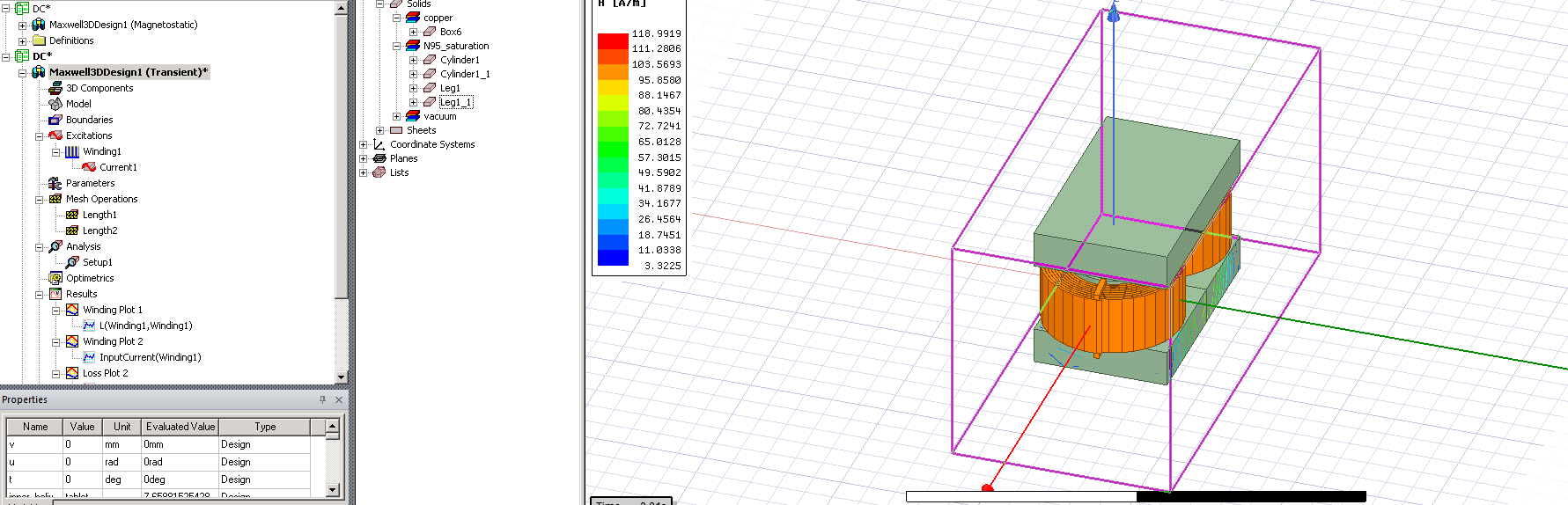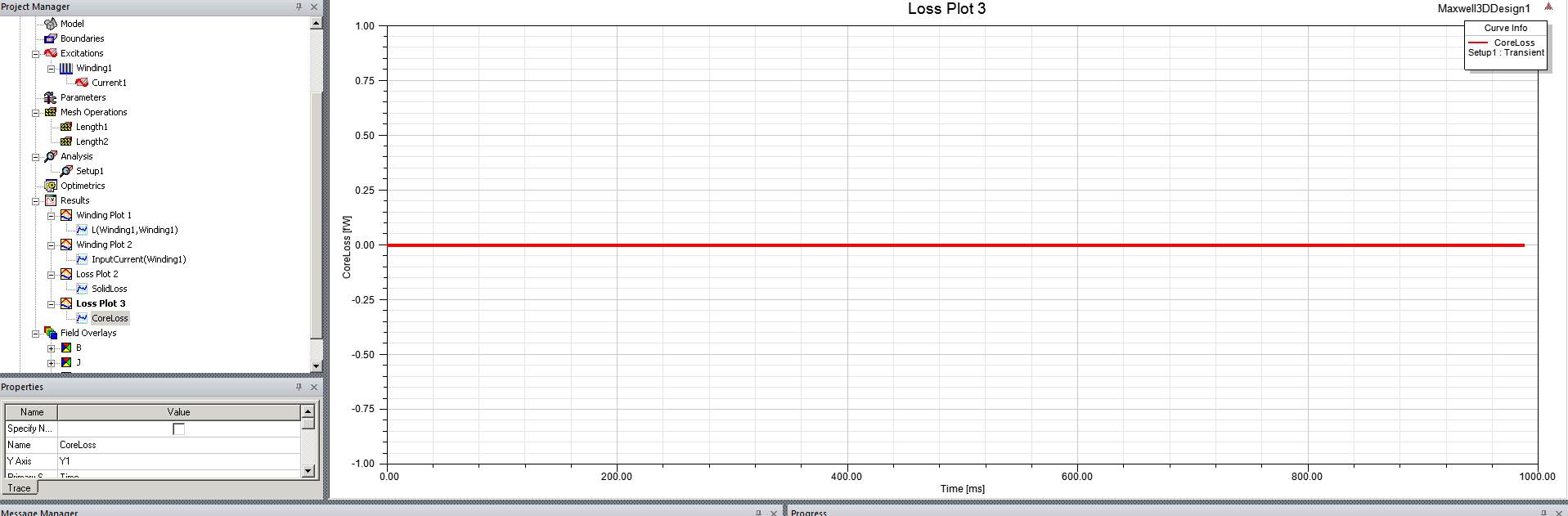## Electronics

#### DC and AC Simulation on Ansys Maxwell for calculating the core and winding losses in an Inductor

Tagged:

•Isha_Engg
Subscriber
I want to simulate the core and the winding losses in an inductor using Ansys Maxwell when imprinted with a triangular pulse(positive RMS Current). I am doing a DC simulation to get the inductance matrix to get an equivalent inductance(To get the ripple current). Then an AC simulation with the current harmonic (3 harmonics)of the triangular pulse.nIs there any way to have a complete automation of the AC And DC losses, such that the simulation is optimized using parameterization? What I want to do on Ansys is: optimize the dimensions of the inductor core and the windings so that I get the lower losses and no saturation in the core. So for each dimension, there is a DC simulation and also AC simulation to calculate all the losses, and accordingly, there is a parametric sweep and we get an optimum configuration of the inductor considering the AC as well as DC losses together.n
•icellb1
Ansys Employee
Hi,nBy using transient solver, you can define a excitation with both DC and AC components. You can define the excitation by add winding, and type in any custom waveform in either voltage or current type excitation. The waveform could be written as something like Vdc+pwl_periodic(dataset, time). The pwl_periodic() function will be your custom AC signal, which should be specified by a user defined dataset. The result losses in transient solver will include both DC and AC losses.n
•Isha_Engg
Subscriber
Hello,nSo here we would need to already know the inductance value of the coil rite? This means we need a DC simulation too, with rms value to get the inductance matrix. Or is there a way to get also the inductance in transient simulation?nRegards,nIshanin
•icellb1
Ansys Employee
Hi,nFor transient solver, you can go to design settings > matrix computation, then check enable to calculate inductances.n
•Isha_Engg
Subscriber
Hello,I try to calculate the core loss, but I get zero core loss. What could be the problem in the simulation? I also generated a correct meshing. nCore loss graph is given as zero:nThere is also a parameter Solid loss. What is this loss?nRegards,Ishanin
•icellb1
Ansys Employee
Hi,nYou can check whether you set core loss calculation by right clicking excitation tab-->set core loss, and then tick the object on which you want to calculate core loss. Solid loss represents the resistive loss of solid conductors, it's calculated on conductors with set eddy effect on or related to a solid type winding or conductor.n
•Isha_Engg
Subscriber
Hello, nI had already set the core loss but I still dont get the results. How is solid loss different from ohmic loss?nRegardsn